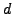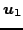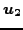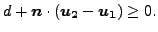Next: *GAP CONDUCTANCE Up: Input deck format Previous: *FRICTION   Contents

## *GAP

Keyword type: model definition

This option is used to define a gap geometry. The parameter ELSET is required and defines the set of gap elements to which the geometry definition applies. Right now, all gap elements must be of the GAPUNI type and can be defined by an *ELEMENT card. The gap geometry is defined by its clearanceand direction(a vector of length 1). Let the displacement vector of the first node of a GAPUNI element beand the displacement vector of the second node. Then, the gap condition is defined by:(170)

A *GAP card automatically triggers a geometrically nonlinear calculation, i.e. the NLGEOM parameter on the *STEP card is automatically triggered.

First line:

• *GAP
• Enter the ELSET parameter and its value.

Second line :

• gap clearance
• first component of the normalized gap direction
• second component of the normalized gap direction
• third component of the normalized gap direction

Example:

*GAP,ELSET=E1
0.5,0.,1.,0.


defines a clearance of 0.5 and the global y-axis as gap direction for all gap elements contained in element set E1.

Example files: gap.Next: *GAP CONDUCTANCE Up: Input deck format Previous: *FRICTION   Contents
guido dhondt 2014-03-02